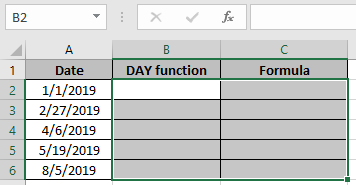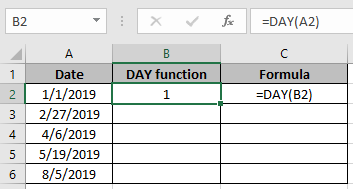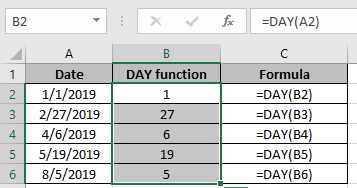# How to Use DAY function in Excel

DAY function in Excel returns just the number from 1-31 corresponding to the date in the argument.

Syntax:

=DAY(date)

Note: Date should be a valid date

Let’s understand this function using the example shown below
Here we have dates. We will use the function on the below shown data set.Use the Formula:

=DAY(A2)

Note: Date should be a valid dateAs you can see in the above snapshot, we have a date as a number from the date.

Copy the formula in the remaining cells to get the new amount for the rest of the data.Date(value) can be extracted using the above procedure

Hope you understood how to use the DAY function in Excel. Explore more articles on Mathematical formulation in Excel here. Mention your queries in the comment box below. We will help you with it.

Related Articles:

How to Use TODAY Function in Excel

How to use the Excel TIME function

How to use the NOW Function in Excel

How to use the WEEKDAY Function in Excel

How to use the MONTH Function in Excel

How to Use the YEAR Function in Excel

Popular Articles:

The VLOOKUP Function in Excel

COUNTIF in Excel 2016

How to Use SUMIF Function in Excel

Terms and Conditions of use

The applications/code on this site are distributed as is and without warranties or liability. In no event shall the owner of the copyrights, or the authors of the applications/code be liable for any loss of profit, any problems or any damage resulting from the use or evaluation of the applications/code.